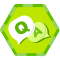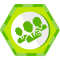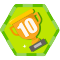Go语言是什么？

Go语言的设计者计划设计一门简单、高效、安全和 并发的语言。这门语言简单到甚至不需要有一个符号表来进行词法分析。它可以快速地编译；整个工程的编译时间在秒以下的情况是常事。它具备垃圾回收功能，因 此从内存的角度是安全的。它进行静态类型检查，并且不允许强制类型转换，因而对于类型而言是安全的。同时语言还内建了强大的并发实现机制。

阅读Go

Go的语法传承了与C一样的风格。程序由函数组成，而函数体是一系列的语句序列。一段代码块用花括号括起来。这门语言保留有限的关键字。表达式使用 同样的中缀运算符。语法上并无 太多出奇之处。

Go语言的作者在设计这一语言时坚持一个单一的指导原则：简单明了至上。一些新的语法构件提供了简明地表达一些约定俗成的概 念的方式，相较之下用C表达显得冗长。而其他方面则是针对几十年的使用所呈现出来的一些不合理的语言选择作出了改进。

变量声明

var sum int // 简单声明
var total int = 42 // 声明并初始化

int* a, b;

var a, b *int

var label = "name"

name := "Samuel"

条件语句

Go语言当中的条件句与C当中所熟知的if-else构造一样，但条件不需要被打包在括号内。这样可以减少阅读代码时的视觉上的混乱。

result := someFunc();
if result > 0 {
/* Do something */
} else {
/* Handle error */
}

if result := someFunc(); result > 0 {
/* Do something */
} else {
/* Handle error */
}

分支语句

int result;
switch (byte) {
case 'a':
case 'b':
{
result = 1
break
}

default:
result = 0
}

var result int
switch byte {
case 'a', 'b':
result = 1
default:
result = 0
}

int result = calculate();
if (result < 0) {
/* negative */
} else if (result > 0) {
/* positive */
} else {
/* zero */
}

switch result := calculate(); true {
case result < 0:
/* negative */
case result > 0:
/* positive */
default:
/* zero */
}

switch result := calculate(); {
case result < 0:
/* negative */
case result > 0:
/* positive */
default:
/* zero */
}

循环

Go只有一个关键字用于引入循环。但它提供了除do-while外C语言当中所有可用的循环方式。

条件

for a > b { /* ... */ }

初始，条件和步进

for i := 0; i < 10; i++ { /* ... */ }

范围

range语句右边的表达式必须是arrayslicestring或者map， 或是指向array的指针，也可以是channel

for i := range "hello" { /* ... */ }

无限循环

for { /* ever */ }

函数

int add(int a, b) { return a + b }

func add(a, b int) int { return a + b }

多返回值

func divide(a, b int) (int, int) {
quotient := a / b
remainder := a % b
return quotient, remainder
}

func divide(a, b int) (quotient, remainder int) {
quotient = a / b
remainder = a % b
return
}

if result, ok := moreMagic(); ok {
/* Do something with result */
}

匿名函数

func makeAdder(x int) (func(int) int) {
return func(y int) int { return x + y }
}

func main() {
}

基本类型

数组和切片

Go语言当中的数组不是像C语言那样动态的。它们的大小是类型的一部分，在编译时就决定了。数组的索引还是使用的熟悉的C语法(如 a[i])，并且与C一样，索引是由0开始的。编译器提供了内建的功能在编译时求得一个数组的长度 (如len(a))。如果试图超过数组界限写入，会产生一个运行时错误。

Go还提供了切片（slices），作为数组的变形。一个切片(slice)表示一个数组内的连续分段，支持程序员指定底层存储的明确部分。构建一个切片 的语法与访问一个数组元素类似：

/* Construct a slice on ary that starts at s and is len elements long */
s1 := ary[s:len]

/* Omit the length to create a slice to the end of ary */
s2 := ary[s:]

/* Slices behave just like arrays */
s == ary[s] //=> true

// Changing the value in a slice changes it in the array
ary[s] = 1
s = 42
ary[s] == 42 //=> true

/* Move the start of the slice forward by one, but do not move the end */
s2 = s2[1:]

/* Slices can only move forward */
s2 = s2[-1:] // this is a compile error

a := [...]int{1,2,3,4,5} // The ... means "whatever length the initializer has"
len(a) //=> 5

/* Slice from the middle */
s := a[2:4] //=> [3 4]
len(s), cap(s) //=> 2, 3

/* Grow the slice */
s = s[0:3] //=> [3 4 5]
len(s), cap(s) //=> 3, 3

/* Cannot grow it past its capacity */
s = s[0:4] // this is a compile error

/* literal */
s1 := []int{1,2,3,4,5}

/* empty (all zero values) */
s2 := make([]int, 10) // cap(s2) == len(s2) == 10

Map类型

m := make(map[string] int) // A mapping of strings to ints

/* Store some values */
m["foo"] = 42
m["bar"] = 30

/* Read, and exit program with a runtime error if key is not present. */
x := m["foo"]

/* Read, with comma-ok check; ok will be false if key was not present. */
x, ok := m["bar"]

/* Check for presence of key, _ means "I don't care about this value." */
_, ok := m["baz"] // ok == false

/* Assign zero as a valid value */
m["foo"] = 0;
_, ok := m["foo"] // ok == true

/* Delete a key */
m["bar"] = 0, false
_, ok := m["bar"] // ok == false

面向对象

Go语言支持类似于C语言中使用的面向对象风格。数据被组织成structs，然后定义操作这些structs的函数。类似于Python，Go语言提供 了定义函数并调用它们的方式，因此语法并不会笨拙。

Struct类型

type Point struct {
x, y float64
}

var p *Point = new(Point)
p.x = 3
p.y = 4

var p1 Point = Point{3,4}  // Value
var p2 *Point = &Point{3,4} // Pointer

方法

func (self Point) Length() float {
return math.Sqrt(self.x*self.x + self.y*self.y);
}

p := Point{3,4}
d := p.Length() //=> 5

/* Note the receiver is *Point */
func (self *Point) Scale(factor float64) {
self.x = self.x * factor
self.y = self.y * factor
}

p.Scale(2);
d = p.Length() //=> 10曾赛2015/06/14
8.8K
8
Kotlin 资源大全 - 学 Kotlin 看这一篇教程就够了

2017/05/19
0
0
11月推荐给程序员们的四本书

2013/11/11
988
2
Android开发技术周报 Issue#154

十一月 10, 20170 条评论AndroidDevWeekly Android开发技术周报 Issue#154 新闻 Google 官方文件管理器 Files Go 上线：入门设备也能流畅运行 Google 发布 Android 十一月更新：修复 ...

2017/11/10
0
0

Primer ：解析结果-不要用。弃用理由：primer.css相当于默认样式，该文件有一大堆标签和类，单位是px和em，我认为在实际开发中会造成类名冲突、px em rem混用影响响应式布局、以及后期反编译...

hhj187
2016/09/04
10
0

Phpstorm2018 永久激活

happyfish319
34分钟前
7
0

###来看一下Android中除了AIDL还有哪些进程间通信的方式： 1、Bundle Bundle实现了Parcelable，所以在Android中我们可以通过Intent在不同进程间传递Bundle数据。 但是在Intent 传输数据的过程...

34分钟前
7
0

Java技术剑
36分钟前
10
0
java 多线程

38分钟前
14
0

42分钟前
7
0